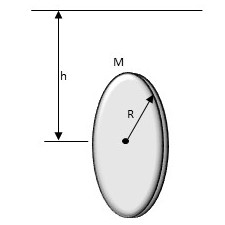# A string is wound around a uniform disk of radius R and Mass M. The disk is released from rest...

## Question:

A string is wound around a uniform disk of radius R and Mass M. The disk is released from rest with the string vertical and the top end tied to a fixed bar.

Show that ( a) the tension in the string is one-third of the weight of the disk and (b) the magnitude of the acceleration of the center of mass is 2g/3.## Torque and Angular Acceleration:

Torque {eq}\tau {/eq} is the rotational equivalent of force. Just as a force is necessary to cause an object to accelerate, torque is necessary to give an object an angular acceleration.

In many cases, when a force is applied to an object it will also create a torque. A force that does not point directly towards (or away from) an object's axis of rotation will also create a torque. In this case, the formula for torque is:

{eq}\vec \tau = \vec r \times \vec F {/eq}

Here, {eq}\vec F {/eq} is the applied force and {eq}\vec r {/eq} is a vector running from the object's axis of rotation to the point where the force was applied. We can also write this formula without vector notation as:

{eq}\tau = rF \sin \theta {/eq}

Here, {eq}\theta {/eq} is the angle between the force and {eq}\vec r {/eq}.

Since torque is the analog of force, we can use it to create an analog of Newton's second law for rotational motion. The linear form of Newton's second law is:

{eq}F_{net} = ma {/eq}

If we replace each quantity with its rotational counterpart, we get:

{eq}\tau_{net} = I\alpha {/eq}

Here, {eq}\alpha {/eq} is the angular acceleration and {eq}I {/eq} is the moment of inertia, which measures an object's resistance to being rotated. The formula for an object's moment of inertia depends on its shape. It may be convenient to have a table of moments of inertia handy when solving physics problems.

Become a Study.com member to unlock this answer! Create your account

(a) This is a Newton's second law problem, but we need to keep track of both forces and torques that act on the disk. Let's begin with force.

What...Torque in Physics: Equation, Examples & Problems

from

Chapter 3 / Lesson 13
32K

After watching this video, you will be able to explain what torque is and use an equation to calculate torque in simple situations. A short quiz will follow.